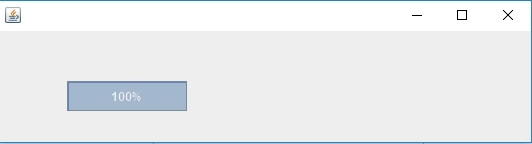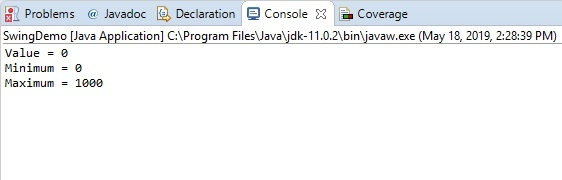# How can we get the values of a JProgressBar Component and display in Console?

Let’s say we have set the following values for JProgressBar −

int min = 0;
int max = 1000;
progressBar = new JProgressBar(min, max);

Now, get the above values and display in the Console −

int value = progressBar.getValue();
System.out.println("Value = "+value);
System.out.println("Minimum = "+progressBar.getMinimum());
System.out.println("Maximum = "+progressBar.getMaximum());

The following is an example to get the values of a progress bar component −

## Example

package my;
import javax.swing.*;
public class SwingDemo extends JFrame {
JProgressBar progressBar;
int i = 0;
SwingDemo() {
int min = 0;
int max = 1000;
progressBar = new JProgressBar(min, max);
int value = progressBar.getValue();
System.out.println("Value = "+value);
System.out.println("Minimum = "+progressBar.getMinimum());
System.out.println("Maximum = "+progressBar.getMaximum());
progressBar.setBounds(70, 50, 120, 30);
progressBar.setValue(0);
progressBar.setStringPainted(true);
setSize(550, 150);
setLayout(null);
}
public void inc() {
while (i <= 1000) {
progressBar.setValue(i);
i = i + 50;
try {
} catch (Exception e) {}
}
}
public static void main(String[] args) {
SwingDemo s = new SwingDemo();
s.setVisible(true);
s.inc();
}
}

This will produce the following output −The following is visible in the Console −